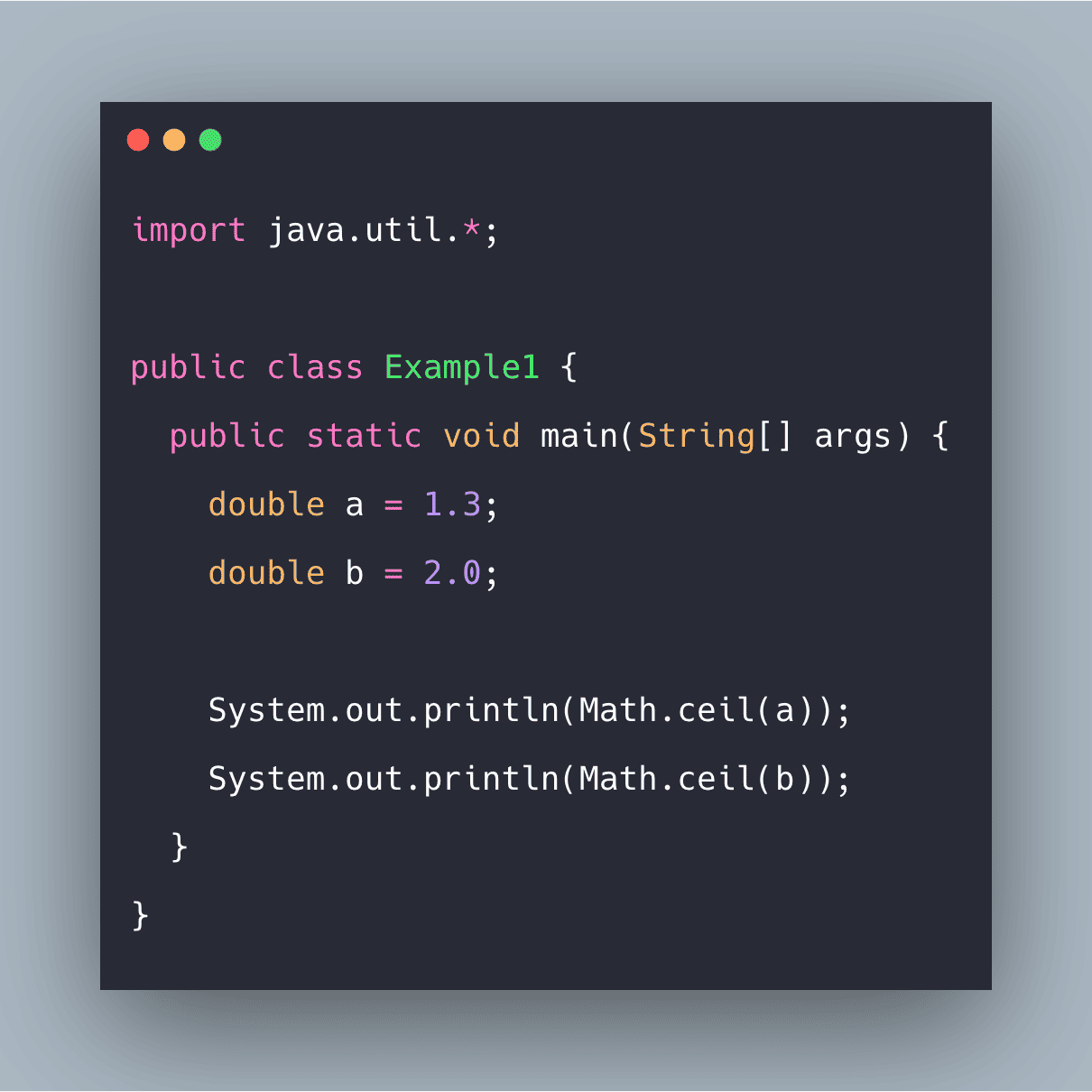# Java Math ceil() Function ExampleJava.lang.Math.ceil() is a method that is used to calculate the nearest integer greater than (or equal to if the passed value is itself an integer) the passed value.

Java.lang.Math.ceil() is an inbuilt method that is used to calculate the nearest integer greater than (or equal to if the passed value is itself an integer) the passed value. Ceil values are often required in various algorithm-building scenarios dealing with mathematical operations and can be directly seen in various mathematical formulae as well.

### Java Math ceil()

Java.lang.Math.ceil(double a) returns the smallest (closest to negative infinity) double value that is greater than or equal to the argument and is equal to a mathematical integer.

1. If the argument value is already equal to a mathematical integer, then the result is the same as the argument.
2. If the argument is NaN or an infinity or positive zero or negative zero, then the result is the same as the argument.
3. If the argument value is less than zero but greater than -1.0, then the result is negative zero.

#### Syntax

``public static double ceil(double x)``

## How to Install OpenJDK 11 on CentOS 8

What is OpenJDK? OpenJDk or Open Java Development Kit is a free, open-source framework of the Java Platform, Standard Edition (or Java SE).

## Math class in Java Example | Java Math Class Tutorial

Math class in Java Example. Using the Math class, we can implement methods like min(), max(), avg(), sin(), cos(), tan(), round(), ceil(), floor(), abs(), pow(), floor(), ciel(), round()

## Java Online Training | Java Online Course | ITGuru

Our Java Online Training provide you to learn about Java programming and its different features with realty. Our Java Online Course includes live sessions, live projects

## Best Java IDE 2020 | Most Popular Java IDE

Our Java Online Training provide you to learn about Java programming and its different features with realty. Our Java Online Course includes live sessions, live projects

## Learning by Doing: How to Learn Java Basics by Building Your Own Project

Check out some ideas for sample projects that can help you learn Java, and programming in general, in a fun and engaging way.